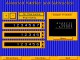Animated Arithmetic 1.0 Teaches addition, subtraction, multiplication and division for 1st - 4th grades. Shareware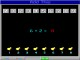Animated Math 1.0 Teaches counting, addition and subtraction with animated rewards. SharewareMath Flash 4.6 Flash card style addition and subtraction practice. SharewareAbilities Builder Whole Number Math Facts 6.1 Learn whole number math facts: addition, subtraction, multiplication, division. Demo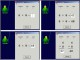Abilities Builder Math Facts 6.6 Learn whole number math facts addition, subtraction, multiplication, division. Freeware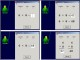Abilities Builder Whole Number Math Facts 7.0 Learn whole number math facts: addition, subtraction, multiplication, division. DemoNotebook Math One 2.4.1 Addition and subtraction . SharewareAbilities Builder Add & Subtract Whole Numbers 6.1 Improve whole number addition and subtraction computation skills. DemoPanIntegers 2.0.1 PanIntegers is a software for the teaching and learning of integers including addition, subtraction, multiplication, division, order of calculations, removal of brackets and simple indices. Shareware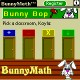BunnyMath (For PalmOS) 1.0 A fun way for children to learn math. Shareware